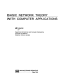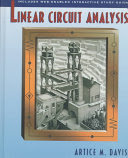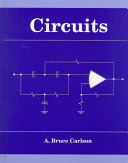### Electrical Circuits

#### Course Description

Basic definitions, terms, clasification, and properties of electrical circuits, elements, modelling, network transformations. Network theorems, electrical signals definition and signal properties, Laplace transform, application to basic signals, application to simple circuits, circuit equations, graphs and networks, definition of branch, node, tree fundamental loop, and cutsets, application ofr graph theory to circuit equations, solution of circuit equations in time and frequency domain, network functions, transfer functions, frequency response, immitance functions, LC and RC immitances, twoports, twoport parameters: z, y, a, h, and g, twoport interconections, basic filter circuits, gain and phase response, electrical transmission lines, definition, time and space signal distribution, reflections.

#### Learning Outcomes

1. Define real electrical component and circuit models.
2. Apply physical laws and mathematical tools for solving el. circuit problems.
3. Use Laplace transform in electrical circuita.
4. Solve electrical circuit using loop, node and state equations.
5. Calculate imitances, transfer functions and characteristic frequencies.
6. Develop passive RLC two-poles.
7. Analyze electrical circuit in time and frequency domains.
8. Analyze and create simple one-, two-ports and electrical filters.
9. Analyze transmission lines and signal transfer.

#### Forms of Teaching

Lectures

Material is tought using Power point presentation and blackboard. Lectures are organized together with laboratory exercises. Lectures also include auditory excercises. Using Moodle system students also solve domestic exercises.

Laboratory

The goal is to introduce electrical components and present measurement equipment and measurement procedures. Individual practical experience in laboratory in applying the concepts that are taught in lectures.

Continuous Assessment Exam
Laboratory Exercises 0 % 15 % 0 % 15 %
Homeworks 0 % 8 % 0 % 8 %
Attendance 0 % 2 % 0 % 2 %
Mid Term Exam: Written 0 % 35 % 0 %
Final Exam: Written 0 % 40 %
Exam: Written 50 % 75 %
##### Comment:

o pass the exam, a student must: -have a minimum of 50% of the points from the middle and final exams, -have a minimum of 50% of the total points, -must complete all 6 laboratory exercises. Possible changes in scoring and grading will be announced on the first lecture.

#### Week by Week Schedule

1. Passive elements: resistors, capacitors, inductors, Modelling of circuit elements: lumped and distributed
2. Kirchhoffs laws, Network transformations: source and immitance transformations, Circuit theorems: superposition, reciprocity, substitution, Tellegen's
3. Active elements: dependent and independent sources, OA, OTA, CCII, CFOA
4. Loops, nodes, modified-nodes equations, Circuit equations in Laplace domain
5. Graphs and networks: nodes, loops, cut-sets, Basics of signal-flow graphs
6. Time and frequency domain equations, Sinusoidal steady-state equations
7. Transfer function, Immitance function
8. Midterm exam
9. Frequency response: gain and phase, Bode plots
10. Single-port circuit synthesis, Properties of RC, RL, and LC immitances
11. Two-port parameters: impedance, admittance, hybrid, transmission, image parameters, Relationships between parameters, Interconnection of two-port circuits
12. Passive RC and RLC filters, Active RC filters
13. Current-mode filters, Primary parameters and transmission parameters
14. Time and space distribution, Reflections
15. Final exam

#### Study Programmes

[FER3-EN] Electrical Engineering and Information Technology - study
(3. semester)

#### Literature

Jurišić, Dražen, Mijat, Neven. (2016.), Električni krugovi-priručnik za studente : Udžbenici Sveučilišta u Zagrebu., Sveučilište u ZagrebuJ. Vlach (1992.), Basic Network Theory with Computer Applications, Van NostrandA. M. Davis (1998.), Linear Circuit Analysis, Brooks/Cole, Pacific Grove, CA, USAA. B. Carlson (.), Circuits, Brooks/Cole, Pacific Grove, CA, USA

#### General

ID 209723
Winter semester
5 ECTS
L3 English Level
L1 e-Learning
60 Lectures
0 Seminar
0 Exercises
12 Laboratory exercises
0 Project laboratory